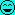# Can You Define Pure vs. Applied Math?

• I
• bballwaterboy
In summary, there is a distinction between pure and applied math, with pure math focused on proving theorems and applied math focused on solving practical problems. This distinction can be seen in various areas of math, and while it may be artificial, it is still widely recognized. However, some believe that this distinction is unnecessary and that pure and applied math should be seen as complementary rather than separate.

#### bballwaterboy

I know in Calculus there is a pure vs. applied calc. distinction. I took Applied Calculus last year, but not the pure form. I've been checking out my university's math catalog and they bring up an applied math vs. pure math track.

I can see that applied math is math that is used in specific settings (chemistry, business, biology, etc.), but I'm still a little uncertain about what is meant by pure math.

Can someone explain the distinction.

Also, does the applied vs. pure distinction only occur when you get to Calculus? What about arithmetic, algebra, pre-calc/trig., geometry, etc. that we learned in high school? Is all the stuff prior to calculus neither applied, nor pure?

TVM!

Hi bbwb:

I think different mathematicians will have different answers. Here is my take.

Pure math is mostly about proving theorems. Examples of areas that are mostly pure math are topology and number theory.

Applied math is mostly about solving practical problems (typically in science and engineering) using mathematical methods. Examples of areas that are mostly applied math are differential equations and linear algebra.

Hope this helps.

Regards,
Buzz

In my experiences this is a typical German distinction. Meanwhile I regard it as a kind of sickness. There have been real camps at my university and each member of one camp used to look down on the members of the other one. The pure mathematicians were sick to hear the question "what for" which they usually hadn't a satisfactory answer to and the applied mathematicians were sick of being seen as "calculators".
PF as a rather good example which shows that this distinction is artificial. To do serious physics you certainly will need knowledge of both.

•Samy_A and micromass
fresh_42 said:
In my experiences this is a typical German distinction. Meanwhile I regard it as a kind of sickness. There have been real camps at my university and each member of one camp used to look down on the members of the other one. The pure mathematicians were sick to hear the question "what for" which they usually hadn't a satisfactory answer to and the applied mathematicians were sick of being seen as "calculators".
PF as a rather good example which shows that this distinction is artificial. To do serious physics you certainly will need knowledge of both.

This is an awesome answer. Sadly, I don't think it is a typical German distinction, it seems to be everywhere. I do agree the distinction is completely artificial. I think that any pure mathematician should have an idea of how pure mathematics is applied in a concrete situation. For example, even a basic knowledge of quantum mechanics reveals a lot about an otherwise pure topic like functional analysis. I believe the converse is true as well.

The work of the pure and the applied mathematician is both interesting and should both be respected. The only distinction is that the pure doesn't care about applications, while the applied always has some application in mind. This does not mean that the pure should not know how his work might be applied. Similarly, the applied mathematician should be aware of any pure advances in his field.

In some respects, the distinction between "applied math" and "pure math" is artificial, with some disciplines starting out as pure mathematics, but becoming applied at a later time. A classic example of this comes from G. H. Hardy's book, "A Mathematician's Apology."

From the wiki page, https://en.wikipedia.org/wiki/A_Mathematician's_Apology:
Some of Hardy's examples seem unfortunate in retrospect. For example, he writes, "No one has yet discovered any warlike purpose to be served by the theory of numbers or relativity, and it seems unlikely that anyone will do so for many years." Since then number theory was used to crack German enigma codes and much later, figure prominently in public-key cryptography.[/quote]

Another example would be high-dimensional vector spaces, which at one time must have been seen as topics in pure math. As it turns out, some manufacturers of CDs and DVDs have applied concepts of 16- and higher-dimensional vector spaces to implement error correction capabilities for recorded music discs.

In addition to the emphasis on proofs, pure math will head toward abstraction. That allows theorems and proofs to concentrate on how much can be proven with the fewest assumptions, trying to get to the root cause of fundamental truths. But it leads them away from specific practical applications. A pure mathematician might be happy if he could prove that a numerical algorithm gave an answer in 100 million function evaluations, but an applied mathematician would not be happy.

Pure math is applied math for the math